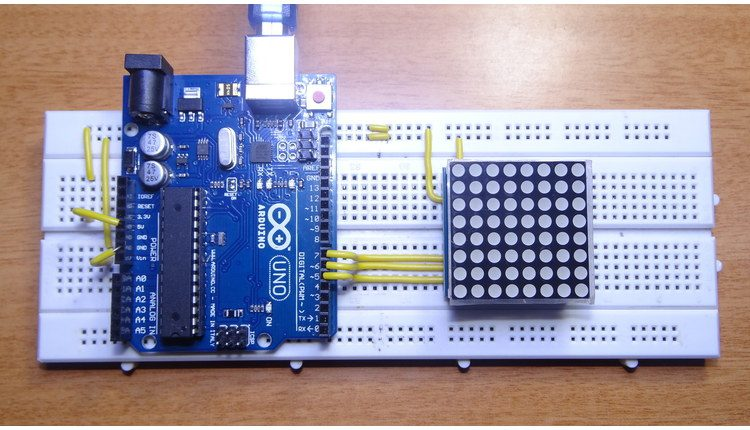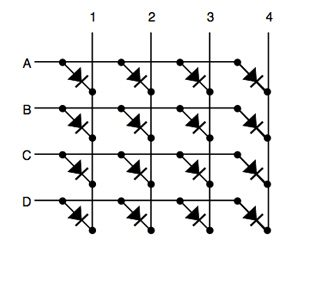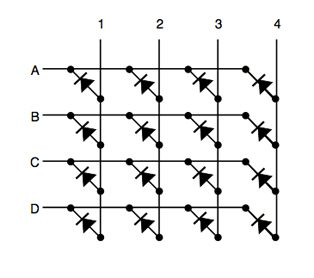# Arduino 8×8 LED Matrix

In this project, we will learn about LED Matrix Displays and two different projects on "Arduino 8×8 LED Matrix Interface". The first project will be a simple interface between Arduino and 8X8 LED Matrix to display information (even scrolling information and images can be displayed) and the second project will be an advanced project where the 8×8 LED Matrix is controlled through an Android device.

An LED matrix is a two dimensional array of LEDs that can be used to display symbols, characters or even images. Based on the orientation of the LEDs in the matrix, there can be two types of LED matrices.They are Common Row Anode and Common Row Cathode.

LED matrix modules are one of the commonly used display devices and are used in major applications like electronic display panels and notification systems.

### Project 1: Simple "Arduino LED Matrix Interface"

#### Circuit Diagram####### Components Required

• Arduino Uno board  [Buy Here]
• LED Matrix – 8 x 8 LED dot matrix
• IC1  – MAX 7219
• R1 – 10 KΩ
• C1 – 0.1 µF
• C2 – 10 µF

#### Component Description

##### Arduino Uno

The project is based on the Arduino Uno microcontroller board. Out of the 14 available digital input / output pins on the Arduino Uno, we need only three pins to implement this project.

One pin provides the clock signal to the LED display driver IC (MAX 7219) while another pin is used to transmit the serial data to the IC for displaying on the LED matrix. The corresponding pins must be appropriately mentioned in the program.

##### LED Matrix

An "8 x 8 LED matrix display" is used in this project to display the information. LED matrices are available in different styles like single color, dual color, multi-color or RGB LED matrix.

They are also available in different dimensions like 5 x 7, 8 x 8, 16 x 16, 32 x 32 etc. Based on the arrangement of the LEDs in the matrix, an LED matrix can be either common row anode or common row cathode.

In case of common row anode type LED matrix, the current sources (high or positive voltage) are given to the rows A-D and the current sinks (low or negative voltage or ground) are given to the columns 1-4.In case of common row cathode type LED matrix, the current sources (high or positive voltage) are given to the columns 1-4 and the current sinks (low or negative voltage or ground) are given to the rows A-D.The LED matrix used in this project is a common row cathode type LED matrix. While developing the project, the type of LED matrix must be known and the program must be written accordingly.

##### IC MAX 7219

The LED matrix can be driven in two ways. They are parallel (where each row or column are sent with parallel data) and serial (where the data is sent serially and an IC is used to convert this serial data into parallel data).

MAX 7219 is a common cathode display driver with serial input and parallel output. It is used to interface microprocessors and microcontrollers with 64 individual LEDs (8 x 8 LED matrix for example has 64 LEDs), seven segment LED displays up to 8 digits or bar graph displays.

The 8 x 8 LED matrix is connected to the MAX 7219 as shown in the circuit diagram and the data input is received from the Arduino board to the MAX 7219.

NOTE:

Pre-wired MAX 7219 and 8 x 8 LED matrix modules are available in the market. They can be used for convenience.#### Working

The aim of the project is to interface an Arduino Uno board with an 8 x 8 LED matrix to display information.

Even though a single 8 x 8 LED matrix with corresponding MAX 7219 IC is used in this project, multiple LED matrices can be connected in series for long scrolling display. Connect the components as shown in the circuit diagram. The working of the system is as follows.

3 of the 14 available digital input / output pins are used to control the display driver IC MAX 7219. The 3 pins on the MAX7219 IC are clock, data in and load (or cs in case of MAX 7221 IC). A maximum clock frequency of 10MHz can be applied. DIN (Data in) accepts the serial data from the microcontroller or Arduino board.

It is 16 bit long where the first 8 bits (D0 – D7) are for driving the columns (SEG A-G and DP of the MAX 7219 IC) of the LED matrix and the next 8 bits (D8 – D15) are for driving the (DIG 0-7 of the MAX 7219 IC) rows of the LED matrix.

The load pin (or CS or chip select pin in case of Max 7221 IC) latches the serial input data on its rising edge.

Another important pin on MAX 7219 is the ISET, which sets the peak current to the segment to drive all the LEDs. It is connected via a resistor (R1), which is called RSET. The capacitors filters out any noise in the supply.

When the serial data in is sent using the Arduino (through the program), the serial data is converted into segments and digits to drive columns and rows of the LED matrix. According to the data sent, the corresponding LEDs on the matrix light up and display the message.

The program written here is for scrolling text display. It might be difficult to view long scrolling data on a single 8 x 8 LED matrix. Hence, multiple LED matrices can be chained to form a long matrix.

The no. of MAX 7219 ICs are equal to the no. of 8 x 8 LED matrices. In order to extend the display to multiple LED matrices, the Data OUT (DOUT) pin of the first MAX 7219 must be connected to the Data IN (DIN) pin of the second MAX 7219 IC. This process must be continued for multiple LED matrices.

(The positioning of the LED Matrix in the chain is important. The first LED matrix must be placed at the right of the chain.)

#### Code

 #include
 int DIN = 13;
 int CS = 12;
 int CLK = 11;
 byte E = {0x3C,0x20,0x20,0x3C,0x20,0x20,0x20,0x3C};
 byte L = {0x20,0x20,0x20,0x20,0x20,0x20,0x20,0x3E};
 byte C = {0x1C,0x20,0x20,0x20,0x20,0x20,0x20,0x1C};
 byte T = {0x7C,0x10,0x10,0x10,0x10,0x10,0x10,0x10};
 byte R = {0x38,0x24,0x24,0x28,0x30,0x28,0x24,0x24};
 byte O = {0x1C,0x22,0x22,0x22,0x22,0x22,0x22,0x1C};
 byte N = {0x42,0x62,0x52,0x52,0x4A,0x46,0x46,0x42};
 byte I = {0x38,0x10,0x10,0x10,0x10,0x10,0x10,0x38};
 byte S = {0x1C,0x20,0x20,0x10,0x08,0x04,0x04,0x38};
 byte H = {0x22,0x22,0x22,0x3E,0x22,0x22,0x22,0x22};
 byte U = {0x22,0x22,0x22,0x22,0x22,0x22,0x22,0x1C,};
 byte B = {0x38,0x24,0x24,0x38,0x38,0x24,0x24,0x38};
 byte smile= {0x3C,0x42,0xA5,0x81,0xA5,0x99,0x42,0x3C};
 byte neutral= {0x3C,0x42,0xA5,0x81,0xBD,0x81,0x42,0x3C};
 byte frown= {0x3C,0x42,0xA5,0x81,0x99,0xA5,0x42,0x3C};
 LedControl lc=LedControl(DIN,CLK,CS,1);
 void setup(){
 lc.shutdown(0,false);
 lc.setIntensity(0,5);
 lc.clearDisplay(0);
 }
 void loop()
 {
 printByte(smile);
 delay(1000);
 printByte(neutral);
 delay(1000);
 printByte(frown);
 delay(1000);
 printByte(E);
 delay(1000);
 printByte(L);
 delay(1000);
 printByte(E);
 delay(1000);
 printByte(C);
 delay(1000);
 printByte(T);
 delay(1000);
 printByte(R);
 delay(1000);
 printByte(O);
 delay(1000);
 printByte(N);
 delay(1000);
 printByte(I);
 delay(1000);
 printByte(C);
 delay(1000);
 printByte(S);
 delay(1000);
 lc.clearDisplay(0);
 delay(1000);
 printByte(H);
 delay(1000);
 printByte(U);
 delay(1000);
 printByte(B);
 delay(1000);
 lc.clearDisplay(0);
 delay(1000);
 }
 void printByte(byte character [])
 {
 int i = 0;
 for(i=0;i<8;i++)
 {
 lc.setRow(0,i,character[i]);
 }
 }

NOTE

• The program uses a library called LedControl. This library must be added to the Arduino IDE and the header file LedControl.h must be included in the program.
• The use of this library file is to enable multiple MAX 7219 ICs to be integrated and also provide scrolling text. The library can be downloaded from this link.

arduino projects
arduino uno
arduino kit
arduino nano
arduino vs raspberry pi
arduino starter kit
arduino board
arduino mega
arduino accelerometer
arduino amazon
arduino alternatives
arduino alarm clock
arduino analog output
arduino and raspberry pi
a=arduino matlab
a arduino.cc
a arduino door lock
arduino a technical reference pdf
arduino a/d converter
arduino a/d
arduino a raspberry pi
arduino a excel
arduino bluetooth
arduino button
arduino battery
arduino basics
arduino bluetooth controller
arduino button wiring
arduino b-l072z-lrwan1
arduino_b_l4s5i_iot01a
port b arduino
fdx-b arduino
ga6-b arduino
usb b arduino
sph0645lm4h-b arduino
arduino code
arduino camera
arduino controller
arduino code language
arduino cnc
arduino can bus
arduino clock
arduino cost
c arduino programming
c arduino tutorial
c arduino pdf
c arduino library
c arduino array
c arduino string
c arduino programming pdf
c arduino serial
arduino due
arduino drone
arduino display
arduino definition
arduino data logger